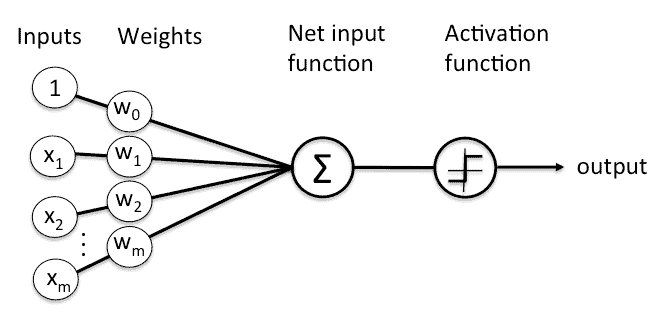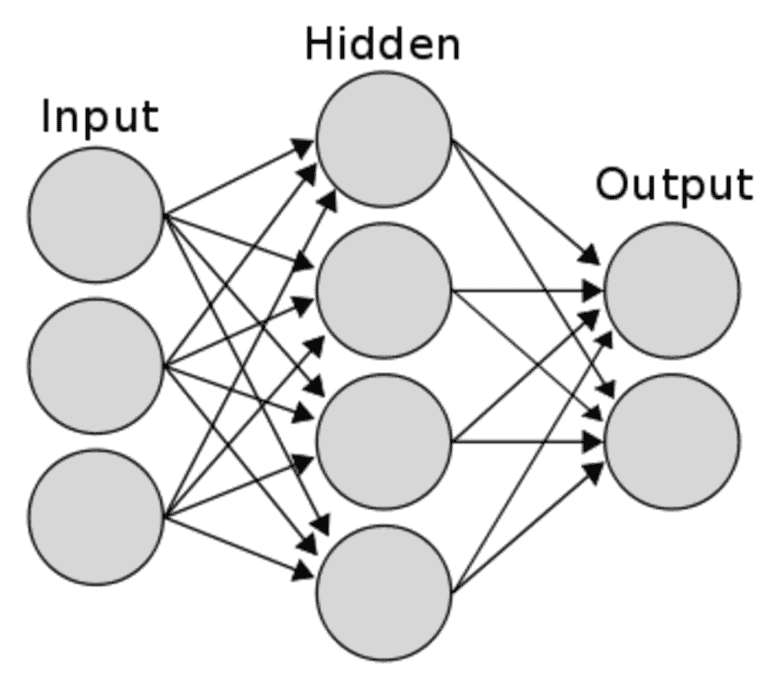## 1. Introduction

In this tutorial, we’ll show a simple explanation for neural networks and their types. Then we’ll discuss the difference between epoch, iteration, and some other terminologies.

## 2. What Is a Neural Network?

### 2.1. Definition

Let’s start with a straightforward example. We have a set of images for dogs and cats. We need a computer application that can learn from these images when to tell if an image is for a dog or cat. So when we come up with a new image the application has never seen, it can tell if the picture has a dog or cat.

In the previous example, we simulate the process of teaching a kid how to be able to differentiate between dogs and cats. This is simply a classification problem, which is one of the most known tasks for neural networks.

Neural Networks are a set of algorithms layered together to recognize the underlying patterns in input data. The patterns they recognize are numerical, contained in vectors, into which all real-world data, be it images, sound, text, or time series.

The neural networks are the brain of deep learning. Deep learning is the scientific and most sophisticated term that encapsulates the “dogs and cats” example we started with.

Applications of neural networks and deep learning are heavily related to image processing, natural language processing, speech recognition, self-driving cars, and robotics.

Like everything else, neural networks have their pros and cons.

### 2.2. Structure

A neural network consists of several layers, each layer consists of set nodes, and the node is where the computations happen.

Any node takes an input vector, along with weights for vector values, to apply some function and send the output to the next layer. The following figure shows a simple node:A set of nodes constructs a layer, and the basic neural network consists of three layers of interconnected nodes; input, hidden, and output. The following figure shows how a simple neural network looks like:### 2.3. Types

Depending on how nodes of each layer are connected, how data is propagated inside the network, how the network learns the patterns, and to what extent the network can remember what it has learned, the name and type of the neural network changes.

There are a bunch of types. Some of these types are; Feed Forward (FF), Recurrent Neural Network (RNN), Long-Short Term Memory (LSTM), and Convolutional Neural Network (CNN).

## 3. Basic Neural Network Terminologies

### 3.1. Epoch

The neural network learns the patterns of input data by reading the input dataset and applying different calculations on it. But the neural network doesn’t make it only once, it learns, again and again, using the input dataset and leaned results from the previous trials.

Each trail to learn from the input dataset is called an epoch.

So an epoch refers to one cycle through the full training dataset. Usually, training a neural network takes more than a few epochs. Increasing the number of epochs doesn’t always mean that the network will give better results.

So basically, by trial-and-error, we choose several epochs at which the results still the same after a very few cycles.

### 3.2. Iteration

For each complete epoch, we have several iterations. Iteration is the number of batches or steps through partitioned packets of the training data, needed to complete one epoch.

### 3.3. Batch

Batch is the number of training samples or examples in one iteration. The higher the batch size, the more memory space we need.

## 4. Differentiate by Example

To sum up, let’s go back to our “dogs and cats” example. If we have a training set of 1 million images in total, it’s a big dataset to feed them all at a time to the network. While training the network, the size of data is keeping to increase in memory. So we’ll divide the dataset into parts or batches.

If we set the batch size to 50K, this means the network needs 20 (1M/50K ) iterations to complete one epoch.

## 5. Conclusion

In this tutorial, we showed the definition, basic structure, and a few types of names of neural networks. Then we showed the difference between epoch, iteration, and batch size.